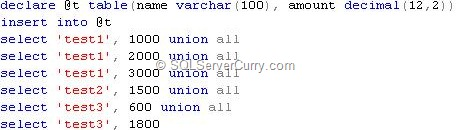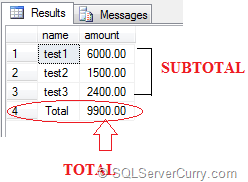# SQL Server: Calculate Summary and Column Summary

This post shows how to show a summary of similar rows as well as column summary for SQL Server table data. Suppose you want to generate the total of a column and display it at the end of the result set. You can use at least two methods as shown below:

Consider the following data:declare @t table(name varchar(100), amount decimal(12,2))
insert into @t
select ‘test1’, 1000 union all
select ‘test1’, 2000 union all
select ‘test1’, 3000 union all
select ‘test2’, 1500 union all
select ‘test3’, 600 union all
select ‘test3’, 1800
If you want to want to sum the amount for each name and also show the total of all names
at the end, use any of the following methods
METHOD 1: Using ROLLUP
select coalesce(name,’Total’) as name, SUM(amount) as amount from @t
group by name
with rollup

METHOD 2: Using UNIONALL
select name, SUM(amount) as amount from @t
group by name
union all
select ‘Total’,SUM(amount) from @t

OUTPUT